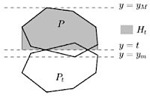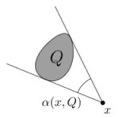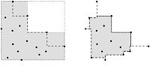Substituting a complex geometric shape by a “simpler” one is motivated by many applications. A typical example is the problem of computing the smallest (area) enclosing disc (or annulus, square, …) of a given set of points in d-dimensional Euclidean space. Unfortunately many of these problems turn out to be computationally difficult. Many of them are NP-hard, and for many others the best known solutions have polynomial runtimes of high degree which renders these algorithms uninteresting from a practical point of view. A possible solution is that instead of insisting on computing the exact solution for such an optimization problem, one looks for a solution that approximates the optimum reasonably well.

#### Inscribing an Axially Symmetric Polygon and other Approximation Algorithms for Planar Convex SetsGiven a planar convex set C, we give sublinear approximation algorithms to determine approximations of the largest axially symmetric convex set S contained in C, and the smallest such set S’ that contains C. More precisely, for any eps>0, we find an axially symmetric convex polygon Q inscribed in C with area |Q|>(1-eps)|S| and we find an axially symmetric convex polygon Q’ containing C with area |Q’|<(1+eps)|S’|.

Hee-Kap Ahn, Peter Brass, Otfried Cheong, Hyeon-Suk Na, Chan-Su Shin and Antoine Vigneron
In Proc. 7th Annual International Computing and Combinatorics Conference, 2004, and in Computational Geometry: Theory and Applications, 33(3):152-164, 2006

#### Aperture-Angle and Hausdorff-Approximation of Convex FiguresThe aperture angle alpha(x, Q) of a point x not in Q in the plane with respect to a convex polygon Q is the angle of the smallest cone with apex x that contains Q. The aperture angle approximation error of a compact convex set C in the plane with respect to an inscribed convex polygon Q of C is the minimum aperture angle of any x in C \ Q with respect to Q. We show that for any compact convex set C in the plane and any k > 2, there is an inscribed convex k-gon Q ofC with aperture angle approximation error (1 – 2/(k+1)) pi. This bound is optimal, and settles a conjecture by Fekete from the early 1990s. The same proof technique can be used to prove a conjecture by Brass: If a polygon P admits no approximation by a sub-k-gon (the convex hull of kvertices of P) with Hausdorff distance sigma, but all subpolygons of P (the convex hull of some vertices of P) admit such an approximation, then P is a (k+1)-gon. This implies the following result: For any k > 2 and any convex polygon P of perimeter at most 1 there is a sub-k-gon Q ofP such that the Hausdorff-distance of P and Q is at most 1/(k+1) * sin(pi/(k+1)).

Hee-Kap Ahn, Sang Won Bae, Otfried Cheong and Joachim Gudmundsson
In Proc. 23rd Annual ACM Symposium on Computational Geometry, pages 37-45, 2007. and to appear in Discrete & Computational Geometry.

#### Maintaining Extremal Points and Its Applications to Deciding Optimal OrientationsGiven a set of points in the plane, we consider the two non-convex enclosingshapes with the minimum area: the L-shape and the quadrant hull. This paper proposes efficient algorithms of computing each of two shapes enclosing given points with the minimum area over all orientations. The algorithms run in time quadratic in the number of given points by efficiently maintaining the set of extremal points.

Hee-Kap Ahn, Sang Won Bae, Chunseok Lee, Sunghee Choi and Kyung-Yong Chwa
In Proc. 18th Annual International Symposium on Algorithms and Computation, 2007.

#### Covering a Point Set by Two Disjoint RectanglesGiven a set S of n points in the plane, the disjoint two-rectangle covering problem is to find a pair of disjoint rectangles such that their union contains S and the area of the larger rectangle is minimized. In this paper we consider two variants of this optimization problem: (1) the rectangles are free to rotate but must remain parallel to each other, and (2) one rectangle is axis-parallel but the other rectangle is allowed to be in arbitrary orientation. For both of the problems, we present O(n2log n)-time algorithms using O(n) space.

Hee-Kap Ahn and Sang Won Bae
In Proc. 19th Annual International Symposium on Algorithms and Computation, 2008.